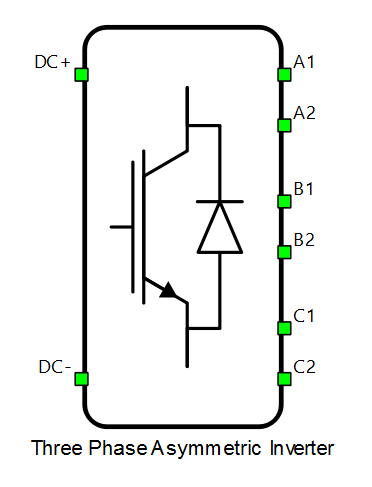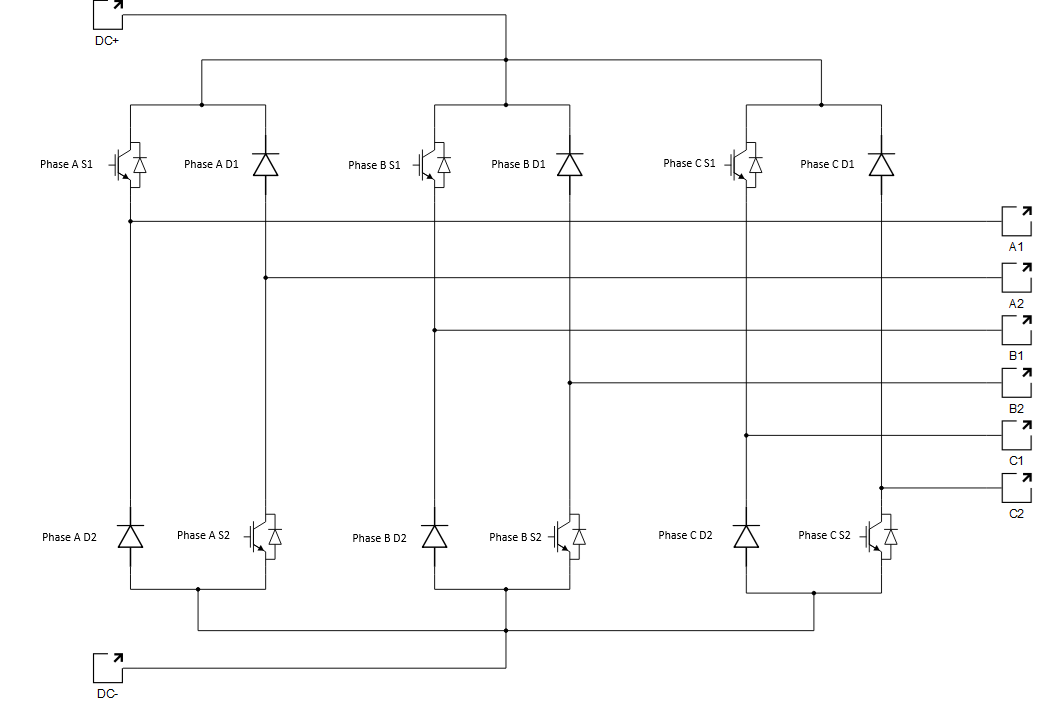# Three phase asymmetric inverter

This section describes three phase asymmetric inverter.

A block diagram and input parameters for the three phase asymmetric inverter block are given in Table 1.

Table 1. Three phase asymmetric inverter component in the Schematic Editor core library
component component dialog window component parametersThree phase asymmetric inverter• Digital input pins for:
• Sa_1 (1..32(64)), Sa_2( (1..32(64))
• Sb_1(1..32(64)), Sb_2( (1..32(64))
• Sc_1(1..32(64)), Sc_2( (1..32(64))
• gate_logic (active high, active low)
Weight =3

Input parameter configuration enables the user to assign gate drive inputs to any of the digital input pins (from 1 to 32). For example, if S1 is assigned to 1, the digital input pin 1 will be routed to the S1 switch gate drive. In addition, the gate_logic parameter selects either active high (High-level input voltage VIH turns on the switch) or active low (Low-level input voltage VIL turns on the switch) gate drive logic, depending on the user’s external controller design.

The schematic block diagram of the three phase asymmetric inverter block is given in Figure 1, with corresponding switch arrangement and naming.Figure 1. The schematic block diagram of a three-phase asymmetric inverter block with corresponding switch naming